# 2 Hemisphere Lighting 半球光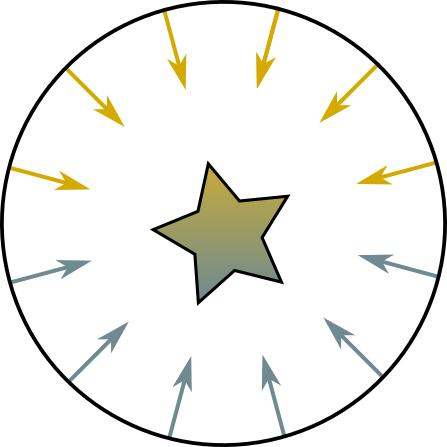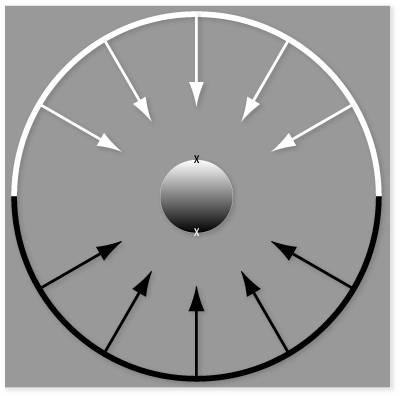Color = a\cdot SkyColor + (1-a)\cdot GroundColor

\begin{array}{c}
a = 1.0 - (0.5\cdot \sin (\theta ) ), \theta \le 90^{\circ} \\
a = 0.5 \cdot \cos (\theta ),\theta \ge 90^{\circ} \\
\end{array}

\theta为法线与光源入射方向的夹角，如下图所示。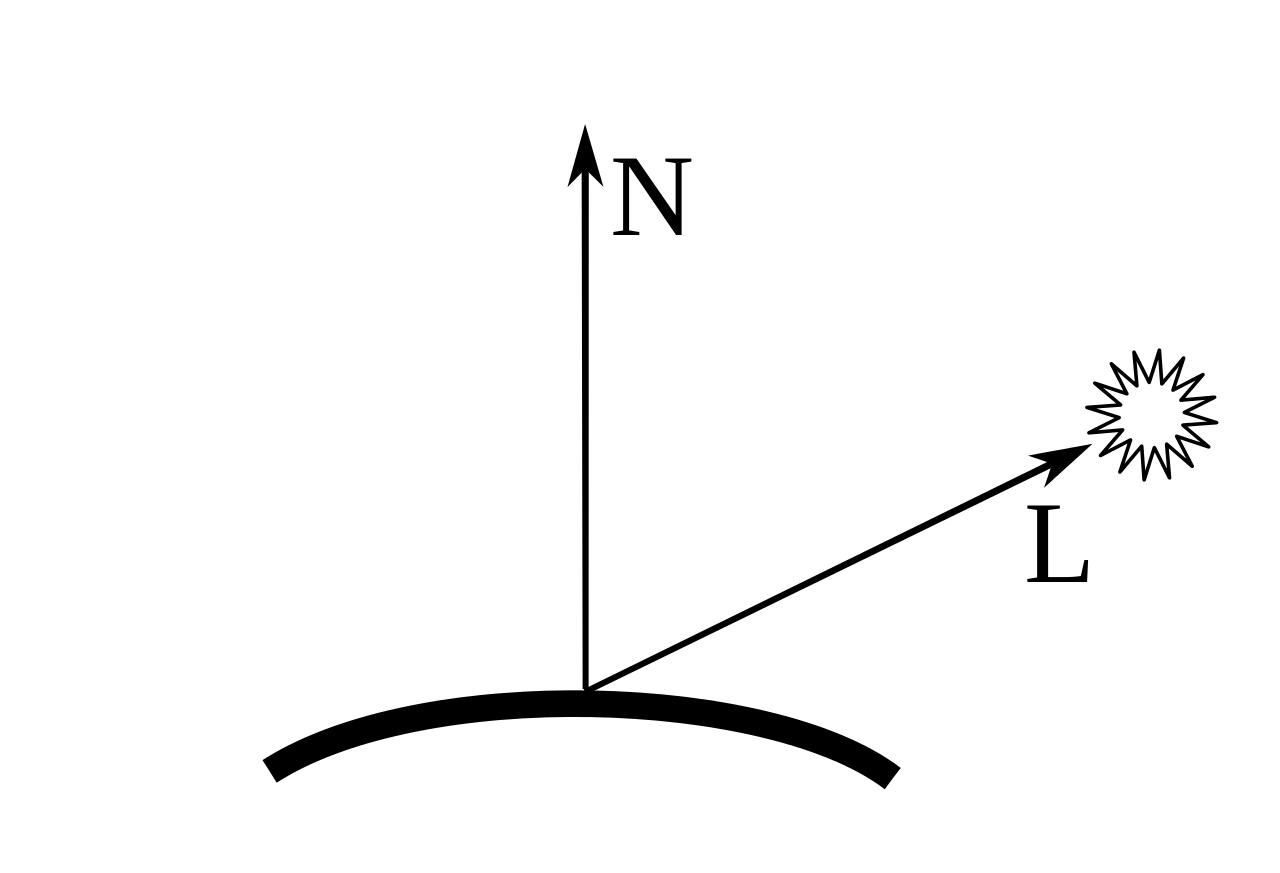a = 0.5 + 0.5 \cdot \cos (\theta )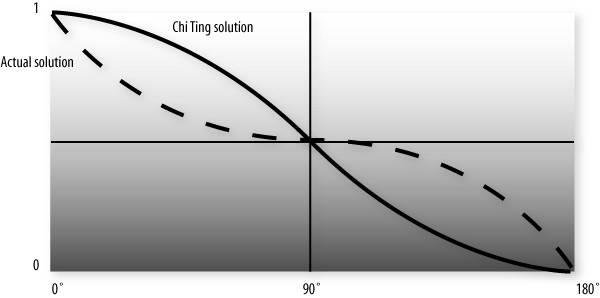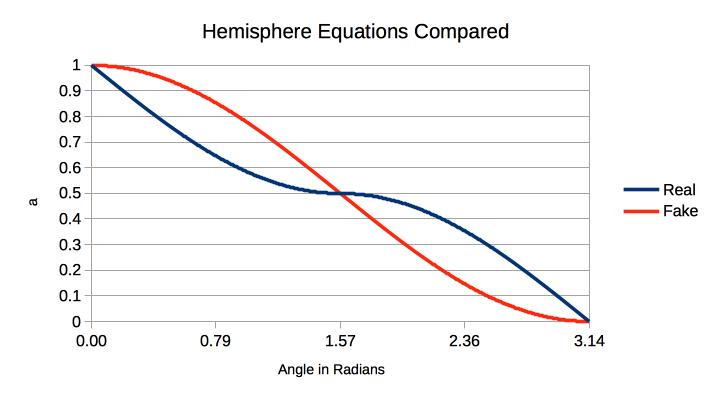uniform vec3 LightPosition;
uniform vec3 SkyColor;
uniform vec3 GroundColor;
void main()
{
vec3 ecPosition = vec3(gl_ModelViewMatrix * gl_Vertex);
vec3 tnorm = normalize(gl_NormalMatrix * gl_Normal);
vec3 lightVec = normalize(LightPosition - ecPosition);
float costheta = dot(tnorm, lightVec);
float a = 0.5 + 0.5 * costheta;

gl_FrontColor = mix(GroundColor, SkyColor, a);

gl_Position = ftransform();
}


//hemisphere lighting shader with optional diffuse lighting path

//inputs
attribute vec4 vPosition;
attribute vec3 vNormal;

//outputs
varying vec4 color;

//structs
struct global_ambient
{
vec4 direction;  // direction of top colour
vec4 top;        // top colour
vec4 bottom;     // bottom colour
};

struct _material
{
vec4 diffuse;
};

//uniforms
uniform mat4 p;     // perspective matrix
uniform mat4 mv;    // modelview matrix

uniform _material material; // material properties
uniform global_ambient global;  // global ambient uniform

//globals
vec4 mvPosition; // unprojected vertex position
vec3 N; // fixed surface normal

void main()
{
//Transform the point
mvPosition = mv*vPosition;  //mvPosition is used often
gl_Position = p*mvPosition;

//Make sure the normal is actually unit length,
//and isolate the important coordinates
N = normalize((mv*vec4(vNormal,0.0)).xyz);

//Make sure global ambient direction is unit length
vec3 L = normalize(global.direction.xyz);

//Calculate cosine of angle between global ambient direction and normal
float cosTheta = dot(L,N);

//Calculate global ambient colour
float a = 0.5+(0.5*cosTheta);
color = a * global.top * material.diffuse
+ (1.0-a)* global.bottom * material.diffuse;
color.a = 1.0; //Override alpha from light calculations - only needs to be done once

}